Custom SearchPerimeter and Area The PERIMETER of a triangle is the sum of the lengths of its sides. In less precise terms, this is sometimes stated as "the distance around the triangle." If the three sides are labeled a, b, and c, the perimeter P can be found by the following formula: P = a + b + c The area of a triangle is the space bounded (enclosed) by its sides. The formula for the area can be found by using a triangle which is part of a rectangle. In figure 17-9, triangle ABC is one-half of the rectangle. Since the area of the rectangle is a times b (that is, ab), the area of the triangle is given by the following formula:Written in terms of h, representing height, the formula is:This formula is valid for every triangle, including those with no two sides perpendicular.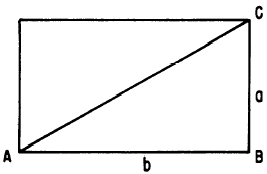Figure 17-9.-Area of a triangle. Practice problems. Find the perimeter and area of each of the triangles in figure 17-10.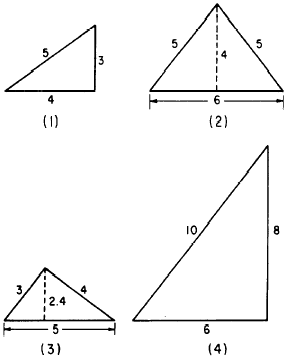Figure 17-10.-Perimeters and areas of triangles. Answers: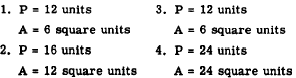CAUTION: The concept of area is meaningless if the units of the multiplied dimensions are not the same. For example, if the base of a triangle is 2 feet long and the altitude is 6 inches long, the area might be carelessly stated as 1/2 (6) (2). However, the units must be considered in order to decide whether the answer is in square feet or square inches. When the units are considered, we realize that the correct answer isSpecial Triangles The classification of triangles depends upon their special characteristics, if any. For example, a triangle may have all three of its sides equal in length; it may have two equal sides and a third side which is longer or shorter than the other two; it may contain a right angle or an obtuse angle. If .it has none of these special characteristics, it is a SCALENE triangle. A scalene triangle has no two of its sides equal and no two of its angles equal. RIGHT TRIANGLE.-If one of the angles of a triangle is a right angle, the figure is a right triangle. The sides which form the right angle are the LEGS of the triangle, and the third side (opposite the right angle) is the HYPOTENUSE. The area of a right triangle is always easy to determine. If the base of the triangle is one of its legs, as in figure 17-10 (4), the other leg is the altitude. If the hypotenuse is acting as the base, as in figure 17-10 (3), the triangle can be turned until one of its legs is the base, as in figure 17-10 (1). If the triangle is not known to be a right triangle, then the altitude must be given, as in figure 17-10 (2), in order to calculate the area. Any triangle whose sides =e in the ratio of 3:4:5 is a right triangle. Thus, triangles with sides as follows are right triangles:(x is any positive number) In addition to the 3-4-5 triangle, two other types of right triangles occur frequently. Any triangle having one 30° angle and one 60° angle is a right triangle; that is, its third angle is 90°. Any triangle having two 45° angles is a right triangle. ISOSCELES TRIANGLE. -A triangle having two of its sides equal in length is an ISOSCE angle, the isosceles triangle has two equal angles. In figure 17-11 (A), triangle ABC is isosceles. Sides AC and BC are equal in length, and angles A and B are equal.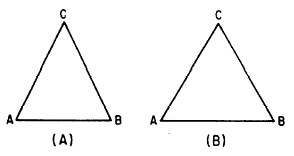Figure 17-11.-(A) Isosceles triangle; (B) equilateral triangle. Figure 17-11 (B) illustrates an EQUILATERAL triangle, which is a special case of an isosceles triangle. An equilateral triangle has all three of its sides equal in length. Since the lengths of the sides are directly related to the size of the angles opposite them, an equilateral triangle is also equiangular; that is, all three of its angles are equal. OBLIQUE TRIANGLES. -Any triangle containing no right angle is an OBLIQUE triangle. Figure 17-12 illustrates two possible configurations, both of which .are oblique triangles. An oblique triangle which contains an obtuse angle is often called an OBTUSE triangle.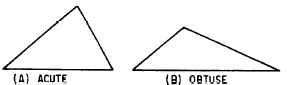Figure 17-12.--Oblique triangles. (A) Acute; (B) obtuse. Sum of the Angles The sum of the angles in any triangle is 180°. For example, if one of the angles is 40° and another is 20°, the third angle is 120°. It is this relationship that justifies the statements made in the preceding section concerning 45° triangles and 30°-60°-90° triangles. If two of the angles are 45° each, then the third angle is 180° - (45°+45°) and the figure is a right tri- angle. If one angle is 60° and another is 30°, the third angle is 90° and the figure is a right triangle.